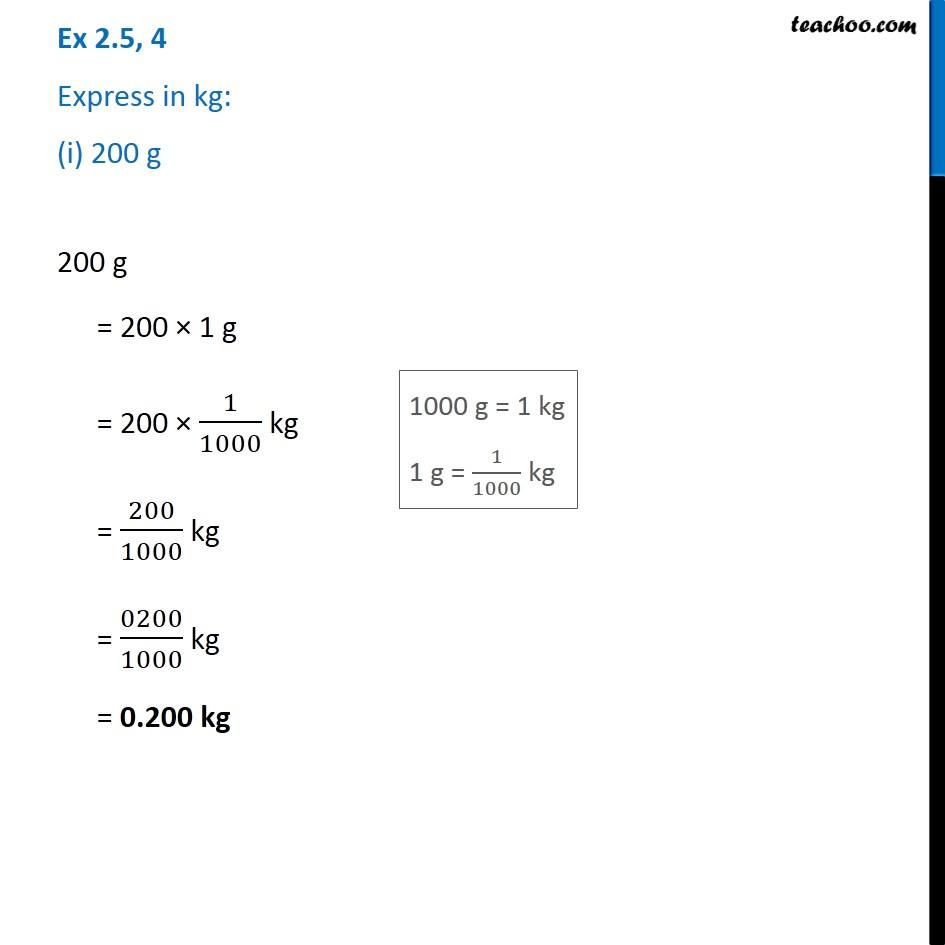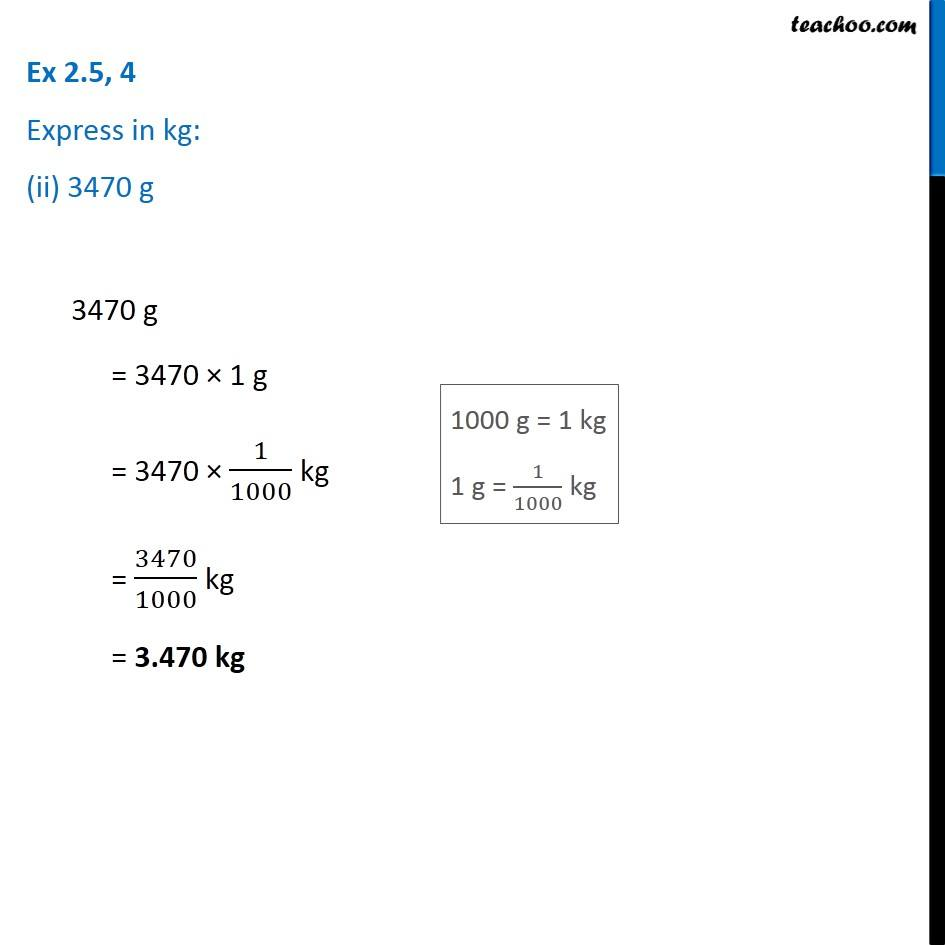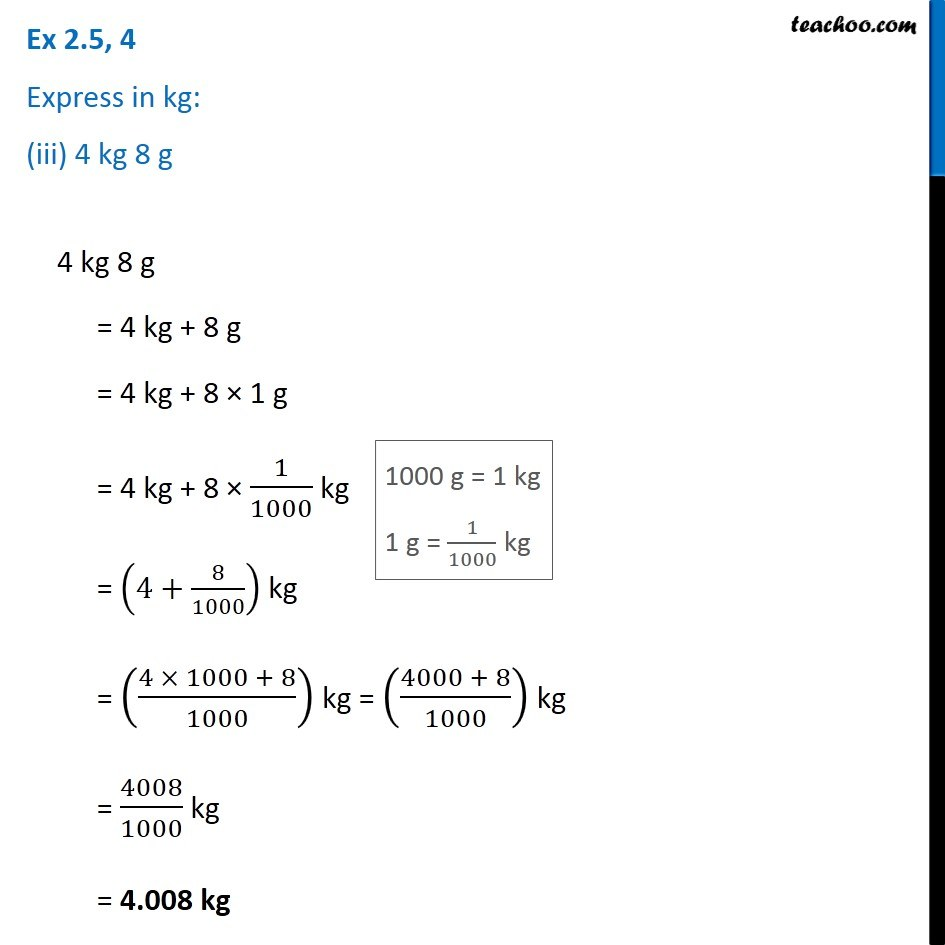Subscribe to our Youtube Channel - https://you.tube/teachoo

1. Chapter 2 Class 7 Fractions and Decimals
2. Concept wise
3. Decimal Numbers

Transcript

Ex 2.5, 4 Express in kg: (i) 200 g 200 g = 200 × 1 g = 200 × 1/1000 kg = 200/1000 kg = 0200/1000 kg = 0.200 kg Ex 2.5, 4 Express in kg: (ii) 3470 g 3470 g = 3470 × 1 g = 3470 × 1/1000 kg = 3470/1000 kg = 3.470 kg Ex 2.5, 4 Express in kg: (iii) 4 kg 8 g 4 kg 8 g = 4 kg + 8 g = 4 kg + 8 × 1 g = 4 kg + 8 × 1/1000 kg = (4+8/1000) kg = ((4 × 1000 + 8)/1000) kg = ((4000 + 8)/1000) kg = 4008/1000 kg = 4.008 kg

Decimal Numbers